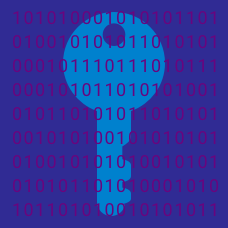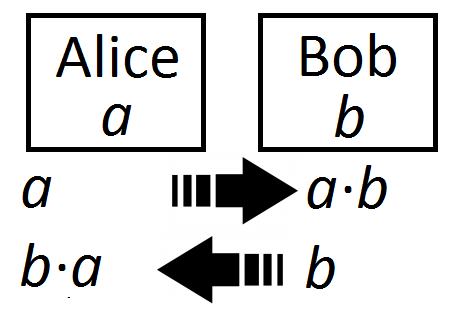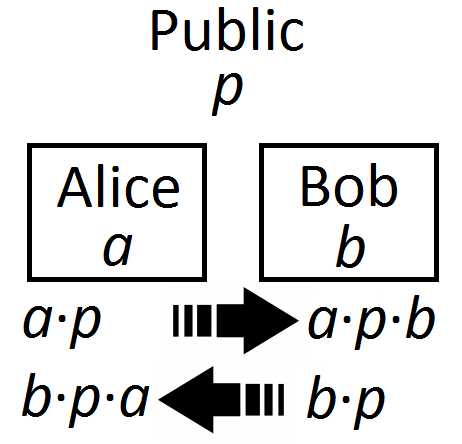Number Theory

# Public-Key EncryptionWhat sort of mathematical breakthrough would endanger the security of the RSA encryption algorithm?

In an encoding scheme, each letter is replaced as follows: $A = 1, \ B = 2, \ C = 3,\ \ldots\ , \ Z = 26.$ For example, $CODE$ would be encoded as $31545,$ since $C = 3,$ $O = 15,$ $D = 4,$ and $E = 5.$

What is the main problem with such a system?

To crack a code, Alice and Bob need to find the product of their secret large primes, $a$ and $b,$ so they each send these primes to each other.Alice receives $b$ and performs $b \cdot a$, while Bob receives $a$ and performs $a \cdot b$.

What's the main issue with this encryption system?

Suppose Alice and Bob want to establish an encryption key. They start by choosing a large prime $p$, which is shared in public.Alice secretly picks a large prime $a$, and Bob secretly picks a large prime $b$. Then both Alice and Bob multiply their primes by $p$, so Alice now has $a \cdot p$ and Bob has $b \cdot p$.

They send $a \cdot p$ and $b \cdot p$ to each other. They then multiply what they receive by what their own secret prime. That is, Alice receives $b \cdot p$ and performs $b \cdot p \cdot a$, and Bob receives $a \cdot p$ and performs $a \cdot p \cdot b$.

Alice and Bob now have the same number that they can use as their secret key.

Is this encryption system secure?

Suppose a cryptography system included the step where, given $m ,$ $e ,$ and $n,$ it is necessary to calculate $c$ in $m^e \equiv c \pmod{n}.$ (That is, first $m^e$ is found, then the value is divided by $n$ with the remainder $c$. )

$e,$ $n,$ and $c$ are going to be shared in public, and it is important that $m$ remains a secret.

Which of these circumstances (if known by someone trying to break the code) would easily compromise $m?$

×